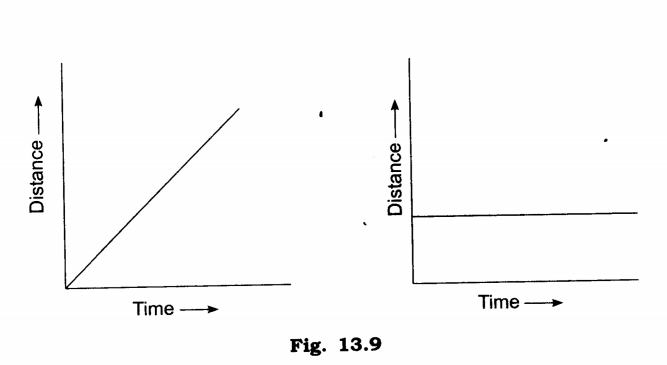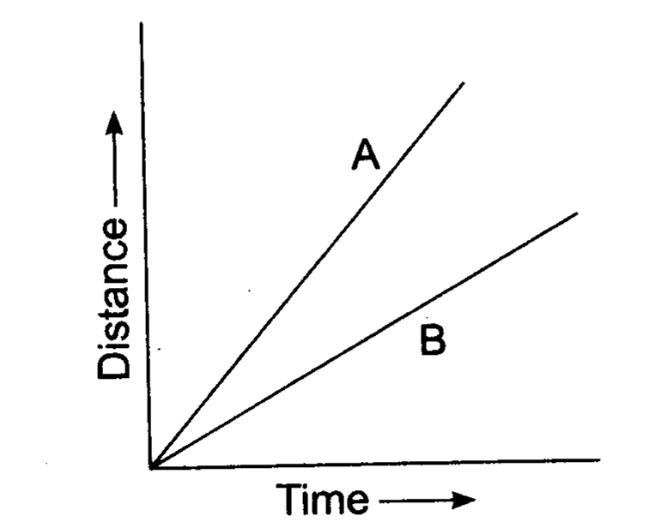## NCERT Solutions Class 7 Science Chapter 13 Motion and Time

Topics and Sub Topics in Class 7 Science Chapter 13 Motion and Time:

 Section Name Topic Name 13 Motion and Time 13.1 Slow or Fast 13.2 Speed 13.3 Measurement of Time 13.4 Measuring speed 13.5 Distance -time graph

Q.1.Classify the following as motion along a straight line, circular or oscillatory motion :
(i) Motion of your hands while running.
(ii) Motion of a horse pulling a cart on a straight road.
(iii) Motion of a child in a merry-go-round. •
(iv) Motion of a child on a see-saw.
(v) Motion of the hammer of an electric bell.
(vi) Motion of a train on a straight bridge.
Ans.(i) Oscillatory motion
(ii) Linear motion
(iii) Circular motion
(iv) Oscillatory motion
(v) Oscillatory motion
(vi) Linear motion

Q.2.Which of the following are not correct?
(i) The basic unit of time is second.
(ii) Every object moves with a constant speed.
(iii) Distances between two cities are measured in kilometers.
(iv) The time period of a given pendulum is not constant.
(v) The speed of a train is expressed in m/h
Ans. (ii), (iv), (v)

Q.3.A simple pendulum takes 32s to complete 20 oscillations, what is the time period of the pendulum?
Ans. Time taken to complete 20 oscillations = 32 s
Time taken to complete 1 oscillation = 32/20 s = 1.6 s
Time period of a pendulum is time taken by it to complete 1 oscillation.
Time period of pendulum is 1.6 seconds.

Q.4.The distance between two stations is 240 km. A train takes 4 hours to cover this distance. Calculate the speed of the train.
Ans.Distance = 240 km
Time taken = 4 hours
Speed =Distance covered/time taken = 240km/4 hammer
=60 km/h
Speed of train =60 km/h

Q.5.The odometer of a car reads 57321.0 km when the clock shows the time 08:30 AM. What is the distance moved by the car, if at 08:50 AM, the odometer reading has changed to 57336.0 km? Calculate the speed of the car in km/min during this time. Express the speed in km/h also.
Ans.Distance = 57336.0 km – 57321 km =15 km
Speed in km/min =15km/20 min = 3/4 km/min

Speed in km/hr =15 km/1/3 hr
=(15 x 3) km/hr
=45 km/hr.

Q.6.Salma takes 15 minutes from her house to reach her school on a bicycle. If the bicycle has a speed of 2 m/min, calculate the distance between her house and the school.
Ans.Time taken =15 min
Speed = 2 m/min .
Distance = speed x time = 2 x 15 = 30 m
Distance between Salma’s school and her house is 30 m.

Q.7.Show the shape of the distance-time graph for the motion in the following cases :
(i) A car moving with a constant speed.
(ii) A car parked on a side road
Ans.Q.8. Which of the following relations is correct?
(i) Speed = Distance Time
(ii) Speed = Distance/Time
(iii)Speed – Time/Distance
(iv)Speed = 1/Distance Time
Ans. (ii) Speed = Distance/Time is correct

Q.9.The basic unit of speed is:
(i) km/min (ii) m/min (iii) km/h (iv) m/s
Ans. (iv) m/s

Q.10.A car moves with a speed of 40 km/h for 15 minutes and then with a speed of 60 km/h for the next 15 minutes. The total distance covered by the car is:
(i) 100 km (ii) 25 km (iii) 15 km (iv) 10 km
Ans.(ii) 25 km
Q.11.Suppose the two photographs, shown in fig. 13.1 and fig. 13.2 of NCERT had been taken at an interval of 10 seconds. If a distance of 100 metres is shown by 1 cm in these photographs, calculate the speed of the blue car.
Ans.0.1 cm/s or 10 m/s

Q.12.Fig 13.10 shows the distance-time graph for the motion of two vehicles A and B. Which one of them is moving faster?Ans. ‘A’ is moving faster.

Q.13.Which of the following distance-time graphs shows a truck moving with speed which is not constant?Ans. (iii)